# GSEB Solutions Class 8 Maths Chapter 4 Practical Geometry InText Questions

Gujarat Board GSEB Textbook Solutions Class 8 Maths Chapter 4 Practical Geometry InText Questions and Answers.

## Gujarat Board Textbook Solutions Class 8 Maths 4 Practical Geometry InText Questions

Try These (Page 58)

Question 1.
Arshad has five measurements of a quadrilateral ABCD. These are AB = 5 cm, ∠A = 50°, AC = 4 cm, BD = 5 cm and AD = 4 cm, BD = 5 cm, and AD = 6cm. Can he construct a unique quadrilateral? Give reasons for answer?
Solution:
No, the quadrilateral ABCD cannot be constructed with the given combination of measurements. Here, knowledge of BC or DC is must.Question 2.
1. We saw that 5 measurements of a quadrilateral can determine a quadrilateral uniquely. Do you think any five measurement of the quadrilateral can do this?
2. Can you draw a parllelogram BATS where BA = 5 cm, AT = 6 cm and AS = 6.5 cm? Why?
3. Can you draw a rhombus ZEAL where ZE = 3.5 cm, diagonal EL = 5 cm? Why?
4. A student attempted to draw a quadrilateral PLAY where PL = 3 cm, LA = 4 cm, AY = 4.5 cm, PY = 2 cm and LY = 6 cm, but could not draw it? What is the reason? [Hint: Discuss it using a rough sketch].
Soution:
1. No, any 5 measurements (elements) cannot determine a quadrilateral. Actually to construct a quadrilateral, we need a specific combination of measurements such as:
(a) Four sides and one diagonal or
(b) Three sides and two diagonals or
(c) Two adjacent sides and three angles or
(d) Three sides and two included angles or
(e) Some special properties are given.

2. Let us draw a rough sketch of BATS as given.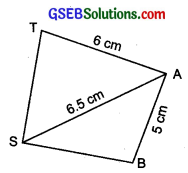Here, we cannot locate the points T and B without knowing the measurements ST and SB respectively.
Thus, we cannot draw a parallelogram with the given measurements.

3. Let us draw a rough sketch of the (rhombus) quadrilateral ZEAL and mark the given measurements on it.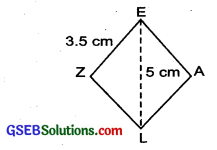Yes, we can draw the required rhombus, because its all sides are equal to 3.5 cm and a diagonal EL is known.

4. Let us draw the rough sketch of the quadrilateral PLAY and mark its measurements,
No, this quadrilateral cannot be drawn.
∵ Point P cannot be located. In ∆LPY, sum of the lengths of PL and PY is less than LY, i.e; (2 cm + 3 cm) < 6 cm.

Try These (Page 62)

Question 1.
“Construct a quadrilateral ABCD, given that BC = 4.5 cm, AD = 5.5 cm, CD = 5 cm, the diagonal AC = 5.5 cm and diagonal BD = 7 cm.” Can we draw the quadrilateral by drawing ∆ABD first and then find the fourth point C?
Solution:
Since, the measurement of AB is not given.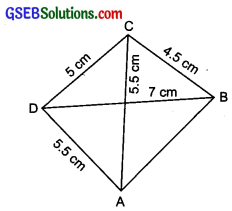∴ We cannot locate the point B. Thus, the quadrilateral cannot be drawn.Question 2.
Can you construct a quadrilateral PQRS with PQ = 3 cm, RS = 3 cm, PS = 7.5 cm, PR = 8 cm and SQ = 4 cm? Justfy your answer?
Solution:
Yes, a quadrilateral PQRS can be drawn using PQ = 3 cm, RS = 3 cm, PS = 7.5 cm, PR = 8 cm and SQ = 4 cm.

Try These (Page 64)

Question 1.
Can you construct the following quadrilateral MIST if we have 100° at M instead of 75°?
Solution:
Yes, the quadrilateral MIST can be constructed with ∠M = 100° instead of 75°.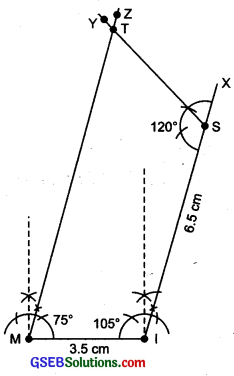Question 2.
Can you construct the quadrilateral PLAN if PL = 6 cm, LA = 9.5 cm, ∠P = 75° ∠L = 150° and ∠A = 140°? (Hint: Recall angle-sum property)
Solution:
Here, ∠p + ∠L + ∠A + ∠N
= 75° + 150° + 140° + ∠N = 365° + ∠N
But the sum of all the angles of a quadrilateral is 360°.
∴ Construction of quadrilateral PLAN is not possible.Question 3.
In a parallelogram, the lengths of adjacent sides are known. Do we still need measures of the angles to construct as in the Q – 1 above?
Solution:
No, the measures of three angles are not necessary in case of a parallelogram as its opposite sides are parallel.

Try These (Page 67)

Question 1.
How will you construct a rectangle PQRS if you know only the lengths PQ and QR?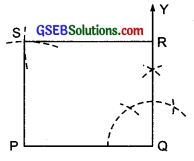Solution:
A rectangle can be constructed by taking PQ as the length making an angle 90° at Q and cutting off QR, the breadth from the ray $$\overrightarrow{Q Y}$$.

Remaining two points R and S can be located by taking P and R as centres and radii as QR and PQ respectively to draw arcs to intersect at S. Thus, PQRS is the required rectangle.Question 2.
Construct the kite EASY if AY = 8 cm, EY = 4 cm and SY = 6 cm. Which properties of the kite did you use in the process?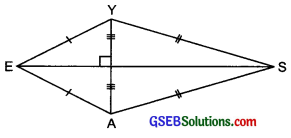Solution:
Following properties have been used in constructing the KITE:

1. Diagonals are at right angles.
2. One of the diagonal bisects the other.
3. Pairs of consecutive sides are equal.

Steps of construction: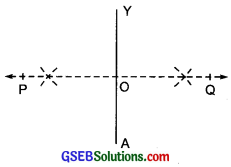I. Draw a line segment AY = 8 cm.
II. Draw $$\overrightarrow{P Q}$$, the perpendicular bisector of AY such that it meets AY at O.
III. We cannot locate a point E on PQ at 4 cm from Y and A, i.e., EY = 4 cm = EA is not possible. It is possible only when E and O coincide. In that case the kite does not exist.